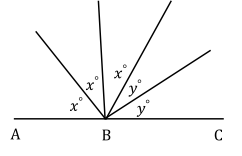# Free SAT Practice Questions

Question 1 of 1
ID: SAT-ATIM-4
Section: Math - Additional Topics in Math
Topics: Geometry; Angles
Difficulty level: Hard

(Practice Mode: Single selected Question » Back to Overview)If ABC is a straight line as shown in the figure above, and $x\$and$\ y$ are integer multiples of 10 with $x>y$, what is the value of $\left(x+y\right)$? (without calculator)